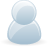# Support Forum

or## RE: Newbie - ADC Pi need circuit help.

2021 Views - Created 08/01/2020

08/01/2020

Posted by:
RTGibsonHi All

I've got a project to do where I need to know the position of a linear actuator - I bought an ADC Pi to help out with the task. I've been lent a test actuator but all the data sheets etc. are in Chinese. basically there's a potentiometer inside the actuator that varies from 0 Ohms (when its retracted) to around 17K (fully extended) - how do I go about hooking this upto one of the inputs on the ADC Pi. It doesn't vary voltage just resistance. Any help with a simple circuit would be massively appreciated.

Many Thanks

Russ

08/01/2020

Posted by:
andrewLocation:
United Kingdom

Hello Russ

The ADC Pi contains a voltage divider on each input consisting of a 10K and 6.8K resistor so the simplest way to measure the position on your linear actuator would be to connect one end of the sensor to the ADC Pi input and connect a stable voltage reference to other end of the sensor. This will in effect change the resistance of the 10K side of the voltage divider between 10K and 27K so you can then measure the voltage on the ADC Pi to get your position.

The schematic below shows how it would work with the ADC Pi voltage divider in the green box and your sensor is the potentiometer at the top.You will need to use a stable voltage reference connected to your sensor as any variations in the input voltage will change the output voltage and in turn your position calculations.

With a 5V reference, the voltage would range between 1.00592V at one end and 2.023V at the other end. The ADC Pi libraries we wrote to work with the board compensate for the voltage divider by multiplying the input voltage by 2.471 so when using the ADC Pi library the voltage should be displayed as 2.485V to 5V.

Our ADC Differential Pi python library also works with the ADC Pi but does not multiply the value by 2.471 so it may be worth using that library for your calculations as it will display the voltage as 1.00592V to 2.023V

If you want to use a different value for the voltage reference we have a voltage divider calculator which will help you with the calculations.

20/01/2020

Posted by:
RTGibsonGreat - thanks for your help.

Russ